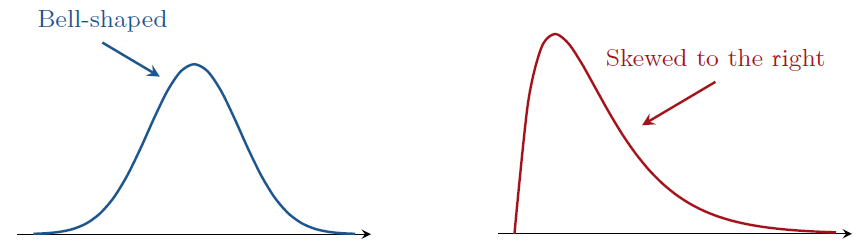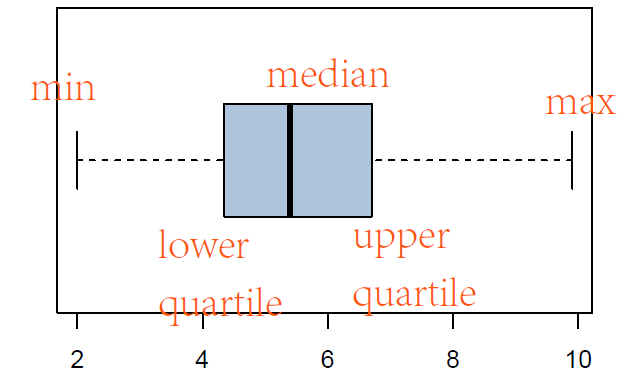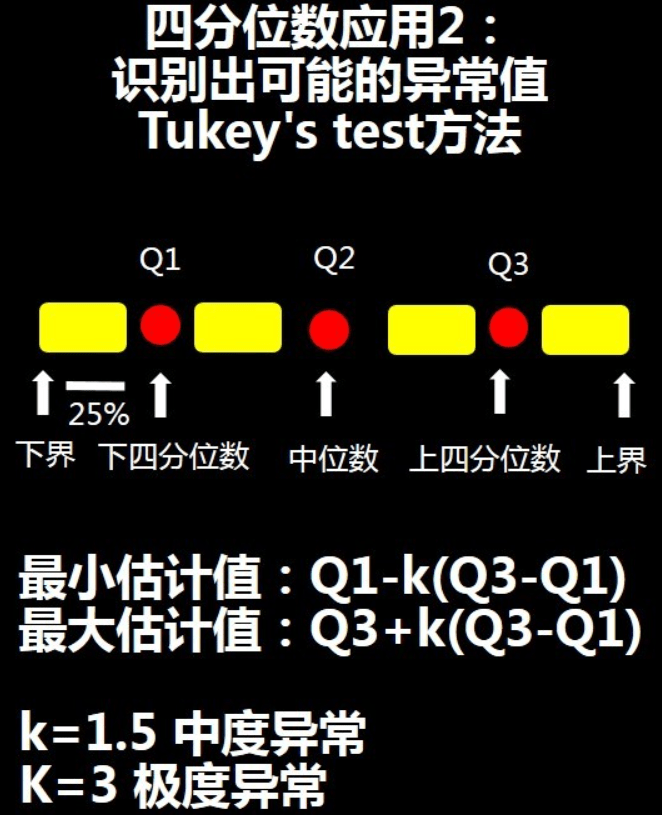# 1. Fundamental concepts in statistics

## 1. Fundamental concepts in statistics

### Frequency Distribution

A frequency distribution is a listing of the possible values for a variable, together with the number, proportion, or percentage of observations at each value. A frequency distribution that has two distinct peaks is called bimodal.

Histogram Graphic: A graph of the frequency distribution of a continuous variable or a discrete variable with intervals of values is called a histogram.

The shape of a histogram is informative.### Describing Data Variability: Standard Deviation and Variance

variability about that center (mean or median)

• The difference between the largest and smallest observations is called the range.

• deviation of observation i of y_i: distances of all the observations from the center (a.k.a. mean or the center of gravity) is (y_i - \overline{y}).

• Standard deviation: For a variable y with n observations y_{1}, y_{2}, \ldots, y_{n} in a sample from some population, the standard deviation s is

s=\sqrt{\frac{\sum_{i=1}^{n}\left(y_{i}-\bar{y}\right)^{2}}{n-1}}=\sqrt{\frac{\left(y_{1}-\bar{y}\right)^{2}+\left(y_{2}-\bar{y}\right)^{2}+\cdots+\left(y_{n}-\bar{y}\right)^{2}}{n-1}} .

​ The standard deviation is the positive square root of the variance s^{2}.

• Variance

s^{2}=\frac{\sum_{i=1}^{n}\left(y_{i}-\bar{y}\right)^{2}}{n-1}### Describing Position: Percentiles, Quartiles, and boxplots

• position: pth percentile
• The pth percentile is the point such that p \% of the observations fall below or at that point and (100-p) \% fall above it. For example, for p=95, the 95 th percentile falls above 95 \% of the observations and below 5 \% of them. The 50 th percentile is the median.

• Quantiles are percentiles expressed in proportion form. For example, the 95 th percentile is also called the 0.95 quantiles.

• lower quartile: the 25 th percentile
• upper quartile: the 75 th percentile
• The quartiles together with the median split the distribution into four parts, each containing one-fourth of the observations.
• The interquartile range, denoted by IQR, is the difference between the upper quartile and the lower quartile.

Unlike the ordinary range, the IQR is not affected by outliers and takes into account variability by observations other than the most extreme ones.（将数据由小到大排序，处于中间的为中位数，即50%分位数，在75%位置的即为75%分位数或四分之三分位数——Q3，在25%位置的即为25%分位数或四分之一分位数——Q1）

basic statistical analysis with pandas

# Carbon dioxide emission in European Nations

import pandas as pd

# data manupulation
Carbon.columns  # Index(['Nation', 'CO2'], dtype='object')
Carbon.tail()   # last 5 rows
Carbon.info()

# find some descriptive statistics
Carbon.describe()   # summary statistics: mean, std, min, max, 25%, 50%, 75%
Carbon['CO2'].mean()    #mean of CO2
Carbon['CO2'].std()     #standard deviation of CO2
Carbon['CO2'].min()     #minimum of CO2
Carbon['CO2'].max()     #maximum of CO2
Carbon['CO2'].median()  #median of CO2

# histogram of CO2
import matplotlib.pyplot as plt
import seaborn; seaborn.set()  # set the style of the plots

plt.hist(Carbon['CO2'], density=True, bins=8)
plt.xlabel('CO2')
plt.ylabel('proportion')
plt.title('Histogram of CO2')

# boxplot
plt.boxplot(Carbon['CO2'], vert=False)